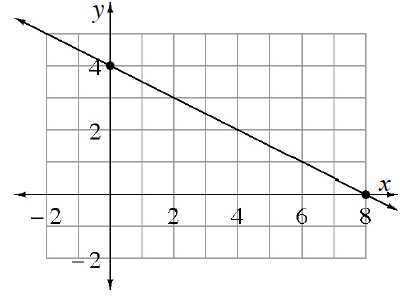### Home > CCG > Chapter 3 > Lesson 3.2.6 > Problem3-111

3-111.This problem is a checkpoint for writing linear equations from multiple representations. It will be referred to as Checkpoint 3.

Write a linear equation that represents each situation. Homework Help ✎

1.An equation for the line at right.

2. An equation for a line perpendicular to the line at right and passing through the point $(−1,−3)$.

3. An equation of the line passing through the points $(4,3)$ and $(−1,1)$.

4. At the concert, Elite Parking charges $\15$ for the first hour and $\7$ for each additional hour of parking. Write an equation to represent the cost $(C)$ for parking $(t)$ hours.

Check your answers by referring to the Checkpoint 3 materials located at the back of your book.

Ideally, at this point you are comfortable working with these types of problems and can solve them correctly. If you feel that you need more confidence when solving these types of problems, then review the Checkpoint 3 materials and try the practice problems provided. From this point on, you will be expected to do problems like these correctly and with confidence.

Answers and extra practice are located in the back of your printed textbook or in the Reference Tab of your eBook.
If you have an eBook for CCG, login and then click the following link: Checkpoint 3: Linear Equations from Multiple Representations